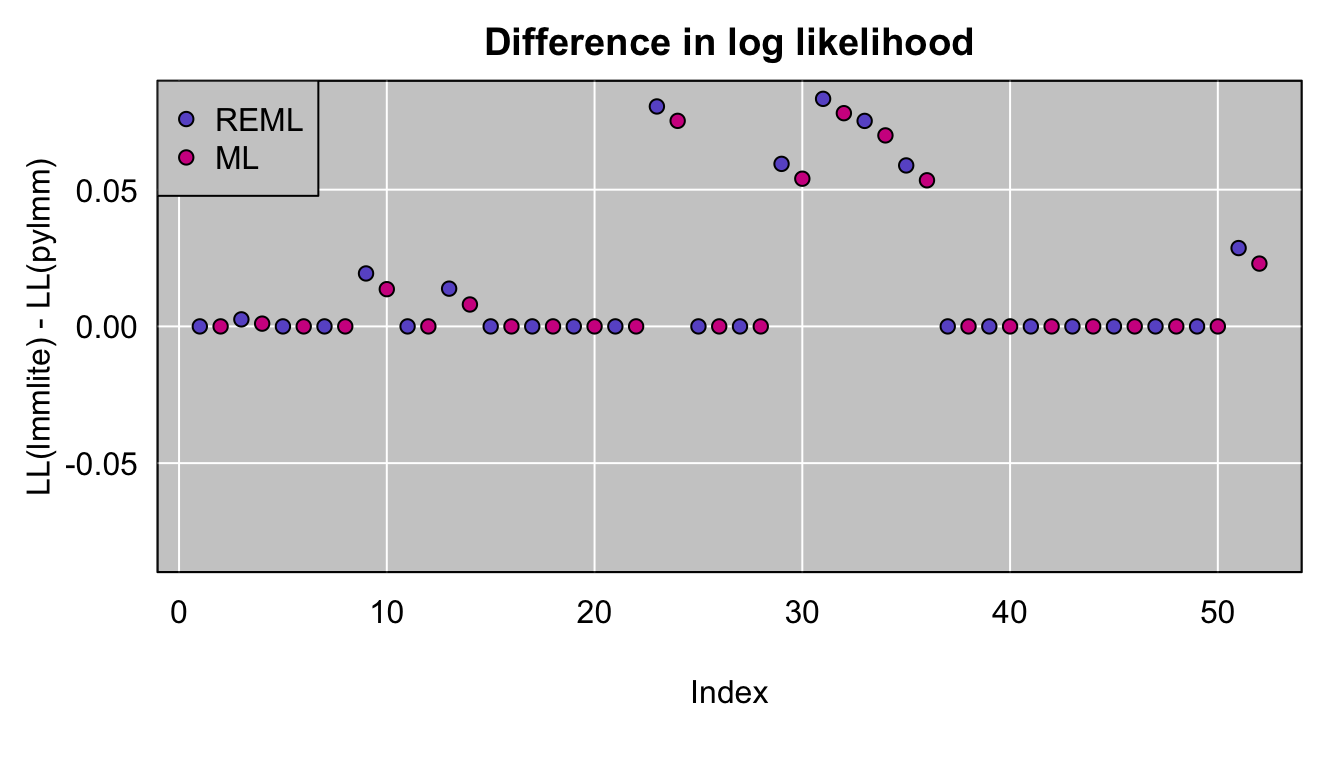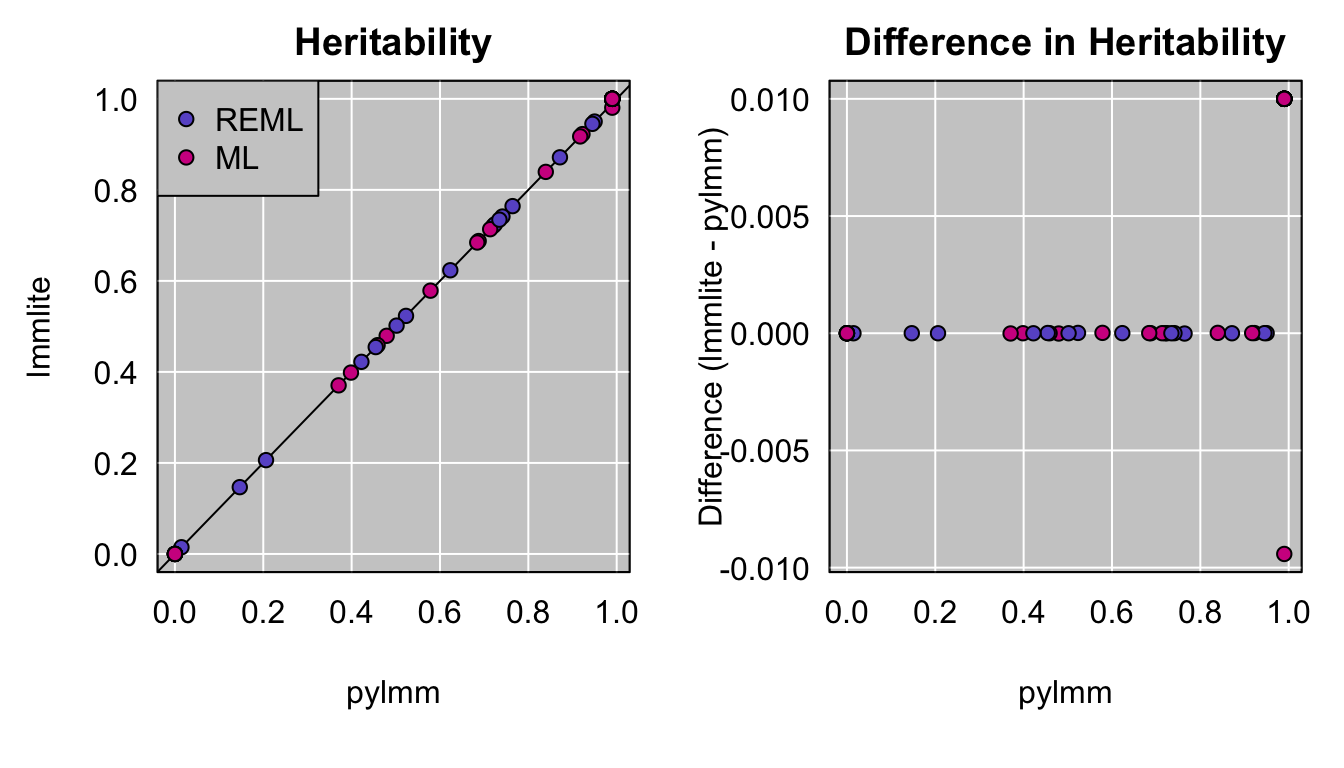My goal with R/lmmlite is a light implementation of the fit of linear mixed models for QTL analysis, to further my understanding of how to do this.

I benefited greatly from Artem Tarasov’s series of blog posts that work through the details of FaST-LMM and pylmm. And I’m closely following Nick Furlotte’s pylmm code, which is remarkably clear and easy to follow.

The sort of model I want to fit is $$y = X\beta + \epsilon$$ where $$\epsilon$$ is multivariate normal with mean 0 and variance $$\sigma^2_g K + \sigma^2_e I$$, where $$K$$ is a known kinship matrix and $$I$$ is the identity. In pylmm, the focus is on $$\sigma^2 = \sigma^2_g + \sigma^2_e$$ and the heritability $$h^2 = \sigma^2_g / \sigma^2$$.

To make sure that my code is correct, here I’ll compare the lmmlite results to those of the R package regress (which, as we’ll see, gives rather different results) and pylmm (and fortunately pylmm and lmmlite results do generally correspond).

The source for this vignette is at GitHub.

I’ll focus on the recla dataset that I include with the package. This is data on a set of diversity outcross mice, from Recla et al. (2014) and Logan et al. (2013). The data are originally from the QTL Archive/Mouse Phenotype Database and I’ve also placed them online in the format used by R/qtl2. I used R/qtl2 to calculate genotype probabilities from the MUGA SNP array data. The data include 261 mice, and form a list with three components: the estimated kinship matrix, a matrix with 26 phenotypes, and a covariate matrix that contains an intercept (all 1’s) and sex (0 for female and 1 for male).

library(lmmlite)
data(recla)

Let’s first run the lmmlite code and fit the LMM for each phenotype, by REML and maximum likelihood (ML). Currently, lmmlite doesn’t deal with missing data at all, so we need to do a bit of extra work: for each phenotype, we drop any individuals with missing phenotypes.

I’ll first create an object to contain the results.

n_phe <- ncol(recla$pheno) lmmlite <- data.frame(index=rep(NA,2*n_phe), method=rep("", 2*n_phe), hsq=rep(NA,2*n_phe), intercept=rep(NA,2*n_phe), sex=rep(NA,2*n_phe), sigmasq=rep(NA,2*n_phe), loglik=rep(NA,2*n_phe), stringsAsFactors=FALSE) Now the actual fitting. The first batch of code omits individuals with missing phenotype. I then call eigen_rotation() which does an eigen decomposition of the kinship matrix and “rotates” the phenotypes and covariates by the transpose of the matrix of eigenvectors. This turns what would be a general least squares problem into a simpler weighted least squares problem. Then I fit the model, first by REML and then by ML, and grab the key results. all_e <- vector("list", n_phe) for(i in 1:n_phe) { keep <- !is.na(recla$pheno[,i])
y <- recla$pheno[keep,i,drop=FALSE] x <- recla$covar[keep,]
k <- recla$kinship[keep,keep] all_e[[i]] <- e <- eigen_rotation(k, y, x) res <- fitLMM(e$Kva, e$y, e$X, reml=TRUE)
lmmlite[i*2-1,1] <- i
lmmlite[i*2-1,2] <- "reml"
lmmlite[i*2-1,-(1:2)] <- c(res$hsq, res$beta, res$sigmasq, res$loglik)

res <- fitLMM(e$Kva, e$y, e$X, reml=FALSE) lmmlite[i*2,1] <- i lmmlite[i*2,2] <- "ml" lmmlite[i*2,-(1:2)] <- c(res$hsq, res$beta, res$sigmasq, res$loglik) } Comparison to pylmm I’ll now run pylmm in the same way. First, I need to save the data to a set of CSV files. for(i in c("kinship", "pheno", "covar")) write.table(recla[[i]], paste0(i, ".csv"), sep=",", quote=FALSE, row.names=FALSE, col.names=FALSE) Now I can run pylmm, writing the output to "pylmm_results.csv". # this chunk is python code import lmm import numpy # load the datasets kinship = numpy.genfromtxt('kinship.csv', delimiter=',') pheno = numpy.genfromtxt('pheno.csv', delimiter=',') covar = numpy.genfromtxt('covar.csv', delimiter=',') # open file for writing f = open('pylmm_results.csv', 'w') # function to print results def print_result(result, index, method): result_arr = [result, result[0,0], result[1,0], result[0,0], result] for j in range(len(result_arr)): result_arr[j] = str(result_arr[j]) f.write(','.join([str(index), method] + result_arr) + '\n') # print header row f.write('index,method,hsq,intercept,sex,sigmasq,loglik\n') # loop over the columns in pheno # fit the LMM by REML or ML and print the results to STDIN for i in range(pheno.shape): result = lmm.LMM(pheno[:,[i]], kinship, X0=covar).fit(REML=True) print_result(result, i+1, 'reml') result = lmm.LMM(pheno[:,[i]], kinship, X0=covar).fit(REML=False) print_result(result, i+1, 'ml') Now I’ll read the data (into R). pylmm <- read.csv("pylmm_results.csv", as.is=TRUE) Log likelihoods The log likelihood values provided by lmmlite are shifted relative to the pylmm ones, because I dropped a few terms that depend only on the sample size. For both REML and ML, the difference should be $$n[\log(2 \pi) + 1 - \log n]/2$$. Let’s make the adjustment and plot the differences. (I’m going to suppress all of the code to make plots; you can see it in the source for this vignette.)The biggest difference in log likelihood, after the adjustment, is 0.08. For the larger discrepancies, lmmlite is giving a larger likelihood value. The points come in pairs (blue for REML, and then pink for ML for the same phenotype). We can see that there are 8 phenotypes with non-negligible differences, and in each case the REML difference is larger than the ML one, and lmmlite is giving larger likelihood values than pylmm. For completeness, let’s look at the log likelihoods from lmmlite calculated at the heritability estimates from pylmm. I use the function calcLL, which takes a specific value (or vector of values) for the heritability. rev_loglik <- rep(NA, 2*n_phe) for(i in 1:n_phe) { e <- all_e[[i]] rev_loglik[i*2-1] <- calcLL(pylmm$hsq[i*2-1], e$Kva, e$y, e$X, reml=TRUE) rev_loglik[i*2] <- calcLL(pylmm$hsq[i*2], e$Kva, e$y, e\$X, reml=FALSE)
}

Actually the results basically don’t change. It’s not even worth making a plot. The largest absolute change in log likelihood was 0.08, while the differences between lmmlite and pylmm log likelihoods are still as large as 5.6.

Heritability

Let’s turn to the parameter estimates. First, the heritability. Here the results are quite similar (maximum absolute difference 0.01).There seem to be very few differences except at the high end, but actually there are 18 points there, where the two heritability estimates differ by about 0.01. These are all cases where pylmm gives estimated heritability exactly 0.99. In 17 cases, lmmlite gives an estimate that is very close to 1; in the other case, lmmlite gives an estimate around 0.98. So I think this just has to do with the optimization near the boundary — that pylmm is not seeking to optimize the likelihood further when the initial heritability estimate is 0.99.

Here’s a plot of the differences by index, and then repeating the plot of the differences in log likelihoods. You can see that these are the same set of 8 phenotypes when these small differences in estimated heritability and also differences in log likelihood, plus another phenotype with a small difference in estimated heritability but a negligible difference in log likelihood.